# Important DI For SBI PO Mains Set – 97

Directions (1- 5): Study the chart carefully to answer the following questions

 Month Temperature Durban Quito Columbus Lisbon Riyadh Jan 20°C 15°C 20°C 22°C 35°C Feb 21°C 16°C 18°C 20°C 30°C Mar 22°C 18°C 16°C 22°C 32°C Apr 25°C 20°C 15°C 25°C 36°C May 28°C 22°C 14°C 18°C 38°C

1. What is the difference between the average temperature of Durban and that of Quito?

(a) 8°C

(b) 11°C

(c) 9°C

(d) 5°C

(e)None of these

e) 5°C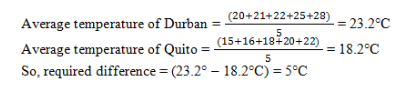2. What is the difference between the average temperature of all cities in May and that if February?

(a) 10°C

(b) 13°C

(c) 3°C

(d) 2°C

(e) None of these

(c) 3°C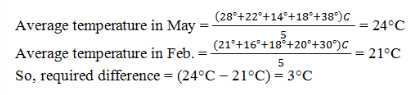3. The average temperature of Riyadh is approximately what percent more than that of Columbus?

(a) 105%

(b) 106%

(c) 93.5%

(d) 87.21%

(e) None of these

(b) 106%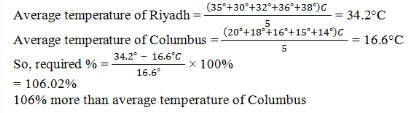4. What is the ratio of the average temperature of Lisbon to that of Quito?

(a) 91 : 88

(b) 107 : 91

(c) 57 : 47

(d) 103 : 95

(b) 107 : 91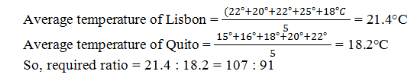5. The average temperature in May is what percent of the average temperature in March of the given five cities?

(a) 89.91%

(b) 103.51%

(c) 120%

(d) 109.09%

(e) None of these

(d) 109.09%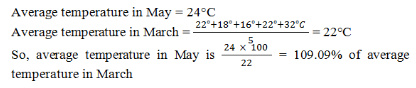Directions (6-10): Study Following table and answer carefully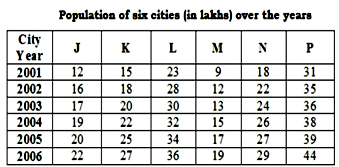6. Total population of city M for all the given years together is what percent of the total population of city P for all the given years together ? (rounded off to nearest integer)

(a) 32

(b) 36

(c) 38

(d) 29

(e) None of these

(c) 38

M = 9+12+13+15+17+19 = 85

P = 31+35+36+38+39+44 = 223

Percentage = (85*100)/223 = 38.11 = 38%

7. What is the respective ratio between total population of cities K, M & N together in 2004 & total population of cities L. J & P together in 2001?

(a) 23 : 25

(b) 21 : 23

(c)21 : 25

(d) 21 : 22

(e)None of these

(d) 21 : 22

K, M & N population in 2004 = 22 + 15 + 26 = 63

L, J & P population in 2004 = 23+12+31 = 66

Ratio = 63: 66 = 21: 22

8. If in 2004 7% of the population of city N was illiterate & 6% of the population of city K was illiterate what is the respective ratio between illiterate populations of cities N & K?

(a) 91 : 68

(b) 89 : 66

(c)92 : 67

(d) 91 : 66

(e) None of these

(d) 91 : 66

N:K = (26*7) : (22*6) = 91: 66

9. Population of city M in 2002 is approximately what percent of the total population of all the six cities in that year ?

(a) 11

(b) 9

(c)14

(d) 7

(e) None of these

(d) 7

Male population in J 2003 = (52*17)/100 = 8.84

Male population in L 2003 = (53*30)/100 = 15.90

Total = 8.84+15.90=24.74 Lakh

10. In 2003. in city J. 48% of the population was female population & for city L it was 47%. What was the total male population (in lakhs) of these two cities in 2003?

(a) 27.44

(b) 23.44

(c) 22.47

(d) 24.74

(e) None of these